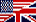PROJECT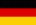RECYCLED  E-THERMOMETER
CONVERTED  INTO   A-V-Ω  - MULTIMETER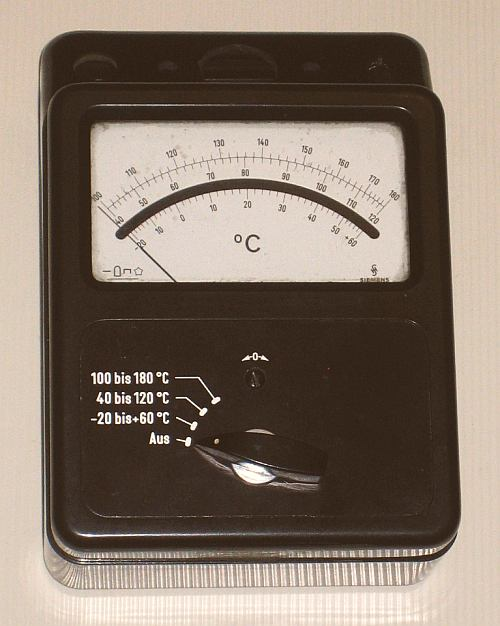```... It starts with this dumped ( analog ) ELECTRICAL THERMOMETER with lost sensor. Because the high-sensitive SIEMENS 20 μA INSTRUMENT is OK, an I D E A came up ... Make a P R O J E C T WITH KIDS - Build a » A-V-Ω MULTI-METER « out of it! =================================================================================== (A) P L A N N I N G : ********************** Use the existing switch for 3 Ranges ... ( _ ) OFF (1st) 12 Volt (2nd) 600 Milli-Ampere (3rd) Ohm ( with 1.5 V BATTERY in box on back side ) On the empty top a T E S T board with PLUGS & POTI will be installed ...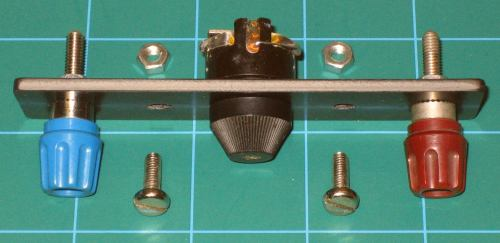How the 20 μA instrument looks INSIDE ...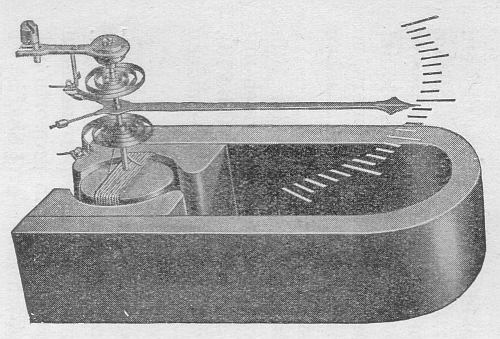The current in the little spool generates a magnetic field, what interact with the magnetic field of the permanent magnet to a torque of the axle. The 2 spirals are both contacts of the spool and provide the counter-moment. The torque is readable via arrow on the scale and proportional to the value of the current in the spool. R E M A R K S : =============== In the F O R M S the LETTERS U, I, R refer to Voltage, Current & Resistance. Their U N I T S are VOLT (= V ), AMPERE (= A ) & OHM (= Ω ). (1st) The VOLT Range: --------------------- Common used batteries are 1.5 V types, as »AAA«, »AA« & »MONO« Cells, 4.5 V & 9 V - Blocks and "combined" 12 V - Blocks or CAR BATTERIES ...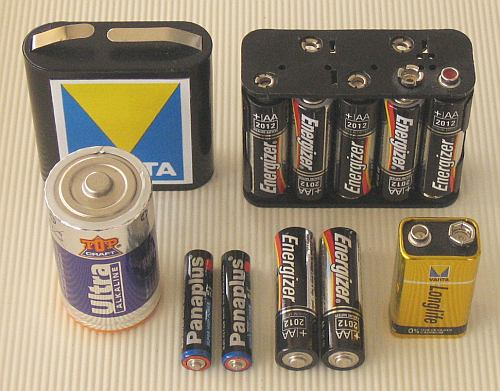With "OHM's LAW" we calculate the (Serial-)RESISTOR (= R ) for a 12 V MAX-Range ... U = R * I ==> R = U / I = 12 V / 20 μA = 600 kΩ Refer to Fig. 1 in the following sketch ...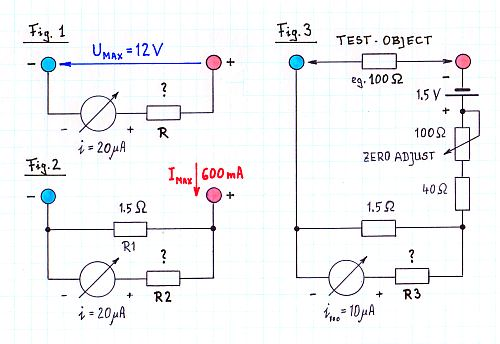(2nd) The AMPERE Range: ----------------------- Common ranges for charging NiCd or NiMH - Cells are 50 mA .. 600 mA. To calculate is the (Parallel-)Resistor (= "SHUNT") for a 600 mA Range of our 20 μA instrument. We have measured: 20 mV = Ri * 20 μA ==> Ri = 1kΩ ... U = Ri * Ii = Rs * Is ==> Rs = Ri * Ii / Is = 1 kΩ * 20 μA / 600 mA = 0.33 Ω This value is too small! It is not realistic to have a smaller shunt than 1.5 Ω (= R1 ). Therefore: We have to make a "Special Arrangement" of resistors ( Ref. to Fig 2 ): Nearly 600 mA go through the shunt R1, 20 μA have to go through the instrument! A (Serial-)Resistor (= R2 ) is neccessary, much bigger than the shunt ... U = R1 * I1 = R2 * I2 ==> R2 = R1 * I1 / I2 = 1.5 Ω * 600 mA / 20 μA = 45 kΩ (3rd) The OHM Range: -------------------- We use the same configuration, add the 1.5 V BATTERY, the TEST-OBJECT-Resistor, and for 0 Ω (= "ShortCut" ) a "ZERO-ADJUST"-Potentiometer of 100 Ω. To limit the "Short-Cut-Current" in 0 Ω Adjustment, an additional 40 Ω resistor is placed serial to the 100 Ω poti. We calculate the value of the (Serial-)Resistor (= R3 ) to place "100 Ω" in the middle of the scale! ( Ref. to Fig 3 ): I = U / R = 1.5 V / ( 100 + 100 ) Ω = ~ 8 mA R3 = 1.5 Ω * ( ~ 8 mA / 10 μA ) = ~ 1.2 kΩ (B) R E A L I S A T I O N : **************************** These theoretical results have to be CONFIRMED and MODIFIED by EXPERIMENTS: (1st) The VOLT Range: --------------------- To reduce swing into its final state, a 20 kΩ resistor ( small compared to R !) is placed parallel to the instrument. R = 570 kΩ was found as the final value. ============ (2nd) The AMPERE Range: ----------------------- As the final value was found R2 = 46 kΩ. ============ (3rd) The OHM Range: -------------------- R3 = 0 Ω was found to be optimal. ========== For safety usage, the V, A & Ω - RANGES get separate COLORED PLUGS ... T H E W I R I N G D I A G R A M : ------------------------------------- Because READABILITY the SCALE-Sequence is A-V-Ω - So the Switch-Sequence too - But the PLUG-Sequence is choosen 0-Ω-A-V because measuring VOLT will be most common ...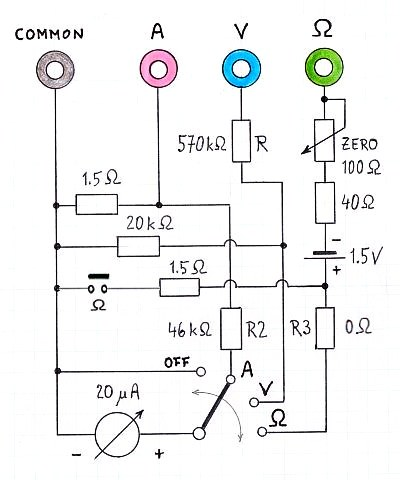... The "OFF" Position is used to "Short-Cut" the instrument for transportation. Moving the instrument generates "Counter-Inductivity" what has a damping effect! R E C Y C L I N G O F T H E E X I S T I N G P R I N T B O A R D ... ----------------------------------------------------------------------------- TOP SIDE BACK SIDE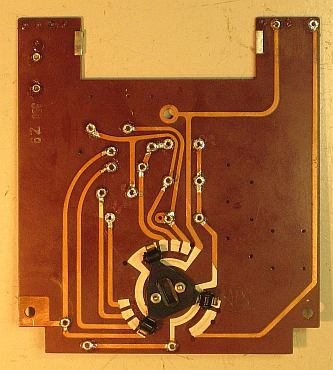... This is another challenge !!! DRAWING THE S C A L E S : -------------------------- Now the SCALES have to be drawn on white cardboard. The V & A Ranges are linear to divide, BUT the Ω-Scale has to be found by experiment ...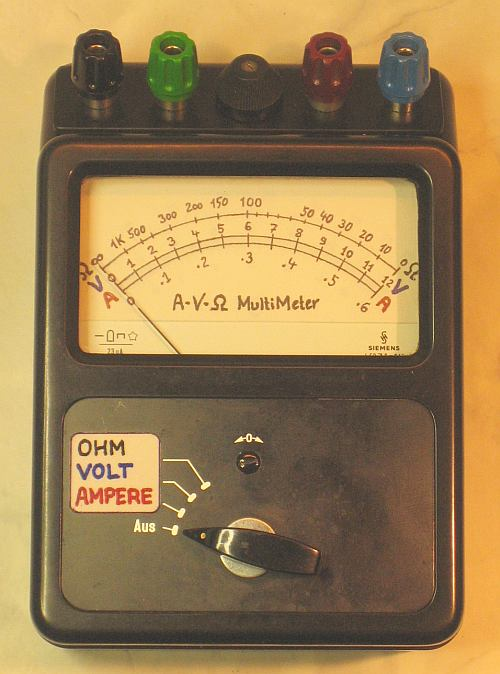This is the final wiring. Bottom (right) shows the battery box with contacts ...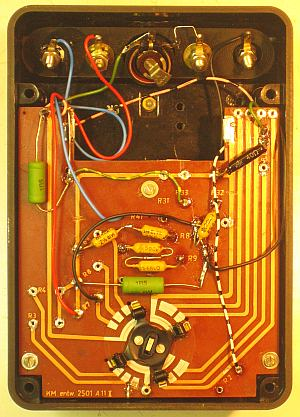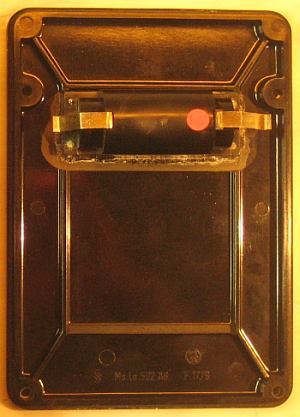... for the 1.5 Volt Battery, a 1/2 of a 3 Volt, eg. VARTA DUPLEX 3010 See in "FAQs" a picture of this BATTERY TYPE !!! impressum: *********************************************************************************** © C.HAMANN http://public.BHT-Berlin.de/hamann 07/19/12 ```